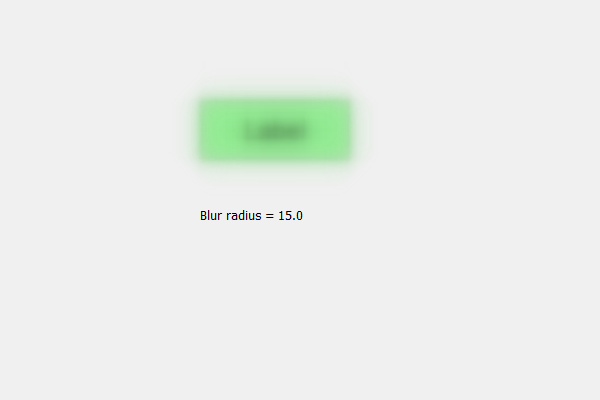# PyQt5 QLabel – Accessing blur radius of the blur effect

In this article we will see how we can access the blur radius of the label blur effect. The blur radius (required), if set to 0 the shadow will be sharp, the higher the number, the more blurred it will be. By default the blur radius value is 5.0 we can change it any time.

In order to do this we use `blurRadius` method.

Here blur_effect is the QGraphicsBlurEffect object

Argument : It takes no argument

Return : It returns foat value

Below is the implementation

 `# importing libraries ` `from` `PyQt5.QtWidgets ``import` `*`  `from` `PyQt5 ``import` `QtCore, QtGui ` `from` `PyQt5.QtGui ``import` `*`  `from` `PyQt5.QtCore ``import` `*`  `import` `sys ` ` `  ` `  `class` `Window(QMainWindow): ` ` `  `    ``def` `__init__(``self``): ` `        ``super``().__init__() ` ` `  `        ``# setting title ` `        ``self``.setWindowTitle(``"Python "``) ` ` `  `        ``# setting geometry ` `        ``self``.setGeometry(``100``, ``100``, ``600``, ``400``) ` ` `  `        ``# calling method ` `        ``self``.UiComponents() ` ` `  `        ``# showing all the widgets ` `        ``self``.show() ` ` `  `    ``# method for widgets ` `    ``def` `UiComponents(``self``): ` ` `  `        ``# creating label ` `        ``label ``=` `QLabel(``"Label"``, ``self``) ` ` `  `        ``# setting geometry to the label ` `        ``label.setGeometry(``200``, ``100``, ``150``, ``60``) ` ` `  `        ``# setting alignment to the label ` `        ``label.setAlignment(Qt.AlignCenter) ` ` `  `        ``# setting font ` `        ``label.setFont(QFont(``'Arial'``, ``15``)) ` ` `  `        ``# setting style sheet of the label ` `        ``label.setStyleSheet(``"QLabel"` `                            ``"{"` `                            ``"border : 2px solid green;"` `                            ``"background : lightgreen;"` `                            ``"}"``) ` ` `  `        ``# creating a blur effect ` `        ``self``.blur_effect ``=` `QGraphicsBlurEffect() ` ` `  `        ``# setting blur radius ` `        ``self``.blur_effect.setBlurRadius(``15``) ` ` `  `        ``# adding blur effect to the label ` `        ``label.setGraphicsEffect(``self``.blur_effect) ` ` `  `        ``# creating a result label ` `        ``result ``=` `QLabel(``self``) ` ` `  `        ``# setting geometry to the result ` `        ``result.setGeometry(``200``, ``200``, ``300``, ``30``) ` ` `  `        ``# getting blur radius ` `        ``b_radius ``=` `self``.blur_effect.blurRadius() ` ` `  `        ``# setting text to the result ` `        ``result.setText(``"Blur radius = "` `+` `str``(b_radius)) ` ` `  `# create pyqt5 app ` `App ``=` `QApplication(sys.argv) ` ` `  `# create the instance of our Window ` `window ``=` `Window() ` ` `  `# start the app ` `sys.exit(App.``exec``()) `

Output :Attention geek! Strengthen your foundations with the Python Programming Foundation Course and learn the basics.

To begin with, your interview preparations Enhance your Data Structures concepts with the Python DS Course.

My Personal Notes arrow_drop_upCheck out this Author's contributed articles.

If you like GeeksforGeeks and would like to contribute, you can also write an article using contribute.geeksforgeeks.org or mail your article to contribute@geeksforgeeks.org. See your article appearing on the GeeksforGeeks main page and help other Geeks.

Please Improve this article if you find anything incorrect by clicking on the "Improve Article" button below.

Article Tags :

Be the First to upvote.

Please write to us at contribute@geeksforgeeks.org to report any issue with the above content.